Lewis Structures

Lewis Structures

The Lewis structure of a compound can be generated by trial and error. We start by writing symbols that contain the correct number of valence electrons for the atoms in the molecule. We then combine electrons to form covalent bonds until we come up with a Lewis structure in which all of the elements (with the exception of the hydrogen atoms) have an octet of valence electrons.

Example: Let's apply the trial and error approach to generating the Lewis structure of carbon dioxide, CO2. We start by determining the number of valence electrons on each atom from the electron configurations of the elements. Carbon has four valence electrons, and oxygen has six.

C: [He] 2s2 2p2

O: [He] 2s2 2p4

We can symbolize this information as shown at the top of the figure below. We now combine one electron from each atom to form covalent bonds between the atoms. When this is done, each oxygen atom has a total of seven valence electrons and the carbon atom has a total of six valence electrons. Because none of these atoms have an octet of valence electrons, we combine another electron on each atom to form two more bonds. The result is a Lewis structure in which each atom has an octet of valence electrons.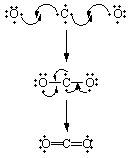A Step-By-Step Approach To Writing Lewis Structures

The trial-and-error method for writing Lewis structures can be time consuming. For all but the simplest molecules, the following step-by-step process is faster.

Step 1: Determine the total number of valence electrons.

Step 2: Write the skeleton structure of the molecule.

Step 3: Use two valence electrons to form each bond in the skeleton structure.

Step 4: Try to satisfy the octets of the atoms by distributing the remaining valence electrons as nonbonding electrons.

The first step in this process involves calculating the number of valence electrons in the molecule or ion. For a neutral molecule this is nothing more than the sum of the valence electrons on each atom. If the molecule carries an electric charge, we add one electron for each negative charge or subtract an electron for each positive charge.

Example: Let's determine the number of valence electrons in the chlorate (ClO3-) ion.

A chlorine atom (Group VIIA) has seven valence electrons and each oxygen atom (Group VIA) has six valence electrons. Because the chlorate ion has a charge of -1, this ion contains one more electron than a neutral ClO3 molecule. Thus, the ClO3- ion has a total of 26 valence electrons.

ClO3-: 7 + 3(6) + 1 = 26

The second step in this process involves deciding which atoms in the molecule are connected by covalent bonds. The formula of the compound often provides a hint as to the skeleton structure. The formula for the chlorate ion, for example, suggests the following skeleton structure.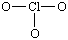The third step assumes that the skeleton structure of the molecule is held together by covalent bonds. The valence electrons are therefore divided into two categories: bonding electrons and nonbonding electrons. Because it takes two electrons to form a covalent bond, we can calculate the number of nonbonding electrons in the molecule by subtracting two electrons from the total number of valence electrons for each bond in the skeleton structure.

There are three covalent bonds in the most reasonable skeleton structure for the chlorate ion. As a result, six of the 26 valence electrons must be used as bonding electrons. This leaves 20 nonbonding electrons in the valence shell.

 26 valence electrons - 6 bonding electrons 20 nonbonding electrons

The nonbonding valence electrons are now used to satisfy the octets of the atoms in the molecule. Each oxygen atom in the ClO3- ion already has two electronsthe electrons in the Cl-O covalent bond. Because each oxygen atom needs six nonbonding electrons to satisfy its octet, it takes 18 nonbonding electrons to satisfy the three oxygen atoms. This leaves one pair of nonbonding electrons, which can be used to fill the octet of the central atom.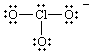Drawing Skeleton Structures

The most difficult part of the four-step process in the previous section is writing the skeleton structure of the molecule. As a general rule, the less electronegative element is at the center of the molecule.

Example: The formulas of thionyl chloride (SOCl2) and sulfuryl chloride (SO2Cl2) can be translated into the following skeleton structures.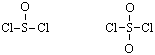It is also useful to recognize that the formulas for complex molecules are often written in a way that hints at the skeleton structure of the molecule.

Example: Dimethyl ether is often written as CH3OCH3, which translates into the following skeleton structure.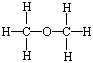Finally, it is useful to recognize that many compounds that are acids contain O-H bonds.

Example: The formula of acetic acid is often written as CH3CO2H, because this molecule contains the following skeleton structure.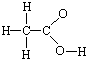Molecules that Contain Too Many or Not Enough Electrons

Too Few Electrons

Occasionally we encounter a molecule that doesn't seem to have enough valence electrons. If we can't get a satisfactory Lewis structure by sharing a single pair of electrons, it may be possible to achieve this goal by sharing two or even three pairs of electrons.

Example: Consider formaldehyde (H2CO) which contains 12 valence electrons.

H2CO: 2(1) + 4 + 6 = 12

The formula of this molecule suggests the following skeleton structure.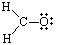There are three covalent bonds in this skeleton structure, which means that six valence electrons must be used as bonding electrons. This leaves six nonbonding electrons. It is impossible, however, to satisfy the octets of the atoms in this molecule with only six nonbonding electrons. When the nonbonding electrons are used to satisfy the octet of the oxygen atom, the carbon atom has a total of only six valence electrons.

We therefore assume that the carbon and oxygen atoms share two pairs of electrons. There are now four bonds in the skeleton structure, which leaves only four nonbonding electrons. This is enough, however, to satisfy the octets of the carbon and oxygen atoms.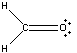Every once in a while, we encounter a molecule for which it is impossible to write a satisfactory Lewis structure.

Example: Consider boron trifluoride (BF3) which contains 24 valence electrons.

BF3: 3 + 3(7) = 24

There are three covalent bonds in the most reasonable skeleton structure for the molecule. Because it takes six electrons to form the skeleton structure, there are 18 nonbonding valence electrons. Each fluorine atom needs six nonbonding electrons to satisfy its octet. Thus, all of the nonbonding electrons are consumed by the three fluorine atoms. As a result, we run out of electrons while the boron atom has only six valence electrons.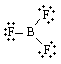The elements that form strong double or triple bonds are C, N, O, P, and S. Because neither boron nor fluorine falls in this category, we have to stop with what appears to be an unsatisfactory Lewis structure.

Too Many Electrons

It is also possible to encounter a molecule that seems to have too many valence electrons. When that happens, we expand the valence shell of the central atom.

Example: Consider the Lewis structure for sulfur tetrafluoride (SF4) which contains 34 valence electrons.

SF4: 6 + 4(7) = 34

There are four covalent bonds in the skeleton structure for SF4. Because this requires using eight valence electrons to form the covalent bonds that hold the molecule together, there are 26 nonbonding valence electrons.

Each fluorine atom needs six nonbonding electrons to satisfy its octet. Because there are four of these atoms, so we need 24 nonbonding electrons for this purpose. But there are 26 nonbonding electrons in this molecule. We have already satisfied the octets for all five atoms, and we still have one more pair of valence electrons. We therefore expand the valence shell of the sulfur atom to hold more than eight electrons.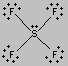This raises an interesting question: How does the sulfur atom in SF4 hold 10 electrons in its valence shell? The electron configuration for a neutral sulfur atom seems to suggest that it takes eight electrons to fill the 3s and 3p orbitals in the valence shell of this atom. But let's look, once again, at the selection rules for atomic orbitals. According to these rules, the n = 3 shell of orbitals contains 3s, 3p, and 3d orbitals. Because the 3d orbitals on a neutral sulfur atom are all empty, one of these orbitals can be used to hold the extra pair of electrons on the sulfur atom in SF4.

S: [Ne] 3s2 3p4 3d0Resonance Hybrids

Two Lewis structures can be written for sulfur dioxide.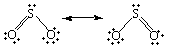The only difference between these Lewis structures is the identity of the oxygen atom to which the double bond is formed. As a result, they must be equally satisfactory representations of the molecule.

Interestingly enough, neither of these structures is correct. The two Lewis structures suggest that one of the sulfur-oxygen bonds is stronger than the other. There is no difference between the length of the two bonds in SO2, however, which suggests that the two sulfur-oxygen bonds are equally strong.

When we can write more than one satisfactory Lewis structure, the molecule is an average, or resonance hybrid, of these structures. The meaning of the term resonance can be best understood by an analogy. In music, the notes in a chord are often said to resonatethey mix to give something that is more than the sum of its parts. In a similar sense, the two Lewis structures for the SO2 molecule are in resonance. They mix to give a hybrid that is more than the sum of its components. The fact that SO2 is a resonance hybrid of two Lewis structures is indicated by writing a double-headed arrow between these Lewis structures, as shown in the figure above.Practice Problem 5:The formula of the amino acid known as glycine is often written as H3N+CH2CO2-. Use the concept of formal charge to explain the meaning of the positive and negative signs in the following Lewis structure.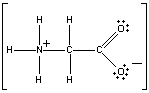Click here to check your answer to Practice Problem 5